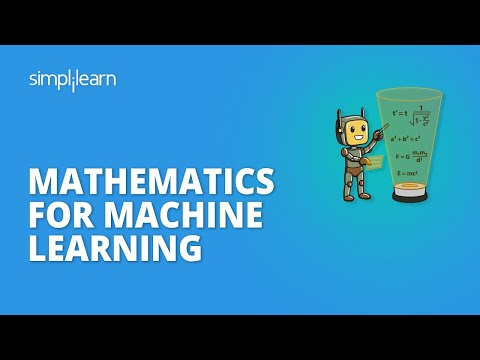Mathematics is one of the most crucial prerequisites for becoming an expert in Machine Learning. It is a foundational skill that you need to possess for working with machine learning algorithms. In this article, you will explore the importance of mathematics for machine learning and cover the skills you need to know in order to enhance your career in machine learning.

You will learn the topics mentioned below, in this article on Mathematics for Machine Learning:

• Importance of Mathematics for Machine Learning
• Statistics and Probability
• Linear Algebra
• Calculus

## Importance of Mathematics for Machine Learning

Expertise in mathematics is necessary to understand and apply algorithms in various applications. From choosing the right algorithm to selecting the correct parameter, it uses mathematical concepts in every step of a machine learning process. Other reasons include choosing an efficient training time, complexity, and bias in variance trade-off.

#### Post Graduate Program in AI and Machine Learning

In Partnership with Purdue UniversityNow, let’s discuss the important skills that you need to know to master mathematics for machine learning.

### 1. Statistics and Probability

Statistics and Probability form the core of data analytics. They are widely used in the field of machine learning to analyze, visualize, interpret data and discover insights. Statistics and probability have found a broad range of applications in several industries. It uses the techniques in statistics and probability theory while collecting, preprocessing, and manipulating data.

Below are some of the topics that you need to know:

• Descriptive Statistics
• Hypothesis Testing
• Regression Analysis
• Probability Distributions
• Conditional Probability
• Sampling and Central Limit Theorem
• Bayes Theorem

Click on the following link to learn more about Statistics and Probability for machine learning: Statistics & Probability for Machine Learning

### 2. Linear Algebra

In the field of machine learning, the concepts of linear algebra come up everywhere. It is another foundation skill required to become an expert in machine learning. To understand how each algorithm works, you need to know linear algebra. Concepts in linear algebra help implement machine learning algorithms from scratch. This includes working with vectors and matrix operations in n-dimensional space.

The following topics are really important:

• Vectors and Matrix Properties
• Matrix Transpose and Inverse
• Determinants
• Dot Product
• Eigenvalues and Eigenvectors
• Matrix Factorization
• Principal Component Analysis
• Orthogonality

Watch the following video to learn the above concepts of Linear Algebra: Linear Algebra for Machine Learning

#### FREE Machine Learning Certification Course

To become a Machine Learning Engineer### 3. Calculus

Knowledge of calculus is very important to understand crucial machine learning applications. You might have to revisit high-school mathematics. Machine learning uses the concepts of calculus to formulate the functions that are used to train algorithms. Machine learning models are trained with datasets having multiple feature variables. Hence, getting familiar with multivariable calculus is important for building a suitable model.

Here are key topics that will help you:

• Differential and Integral Calculus
• Limit, Continuity and Partial derivatives
• Step, Sigmoid, Logit, and ReLU Function
• Maxima and Minima of a Function
• Product and Chain Rule

Here is a video link that will help you understand calculus better: Calculus for Machine Learning

Apart from the above mathematical skills, you should also have good expertise with algorithms and optimization methods. Knowledge of the working of gradient descent algorithms and familiarity with cost function and likelihood function is also critical. Other areas needing to focus on are data structures and discrete mathematics.

Mentioned below are some of the topics you should know:

• Arrays
• Linked List
• Stack
• Queue
• Sorting Algorithms
• Binary Search
• Heap Hashing
• Set and its Properties
• Graph Theory
Looking forward to becoming a Machine Learning Engineer? Check out the Machine Learning Certification Course and get certified today.

## Conclusion

After reading this article, you would’ve understood why mathematics plays a key role in machine learning. Additionally, the skills you need to know, to master machine learning algorithms and build cost-effective models were also made clear. You also learned the different topics that are needed for a better understanding of machine learning.

Do you have questions related to this article on Mathematics For Machine Learning? If you do, then please put them in the comments section. Our team will be happy to help you.

To start your career in machine learning, click on the following link: PG Program in AI and Machine Learning

## About the AuthorAvijeet Biswal

Avijeet is a Senior Research Analyst at Simplilearn. Passionate about Data Analytics, Machine Learning, and Deep Learning, Avijeet is also interested in politics, cricket, and football.

View More
• Disclaimer
• PMP, PMI, PMBOK, CAPM, PgMP, PfMP, ACP, PBA, RMP, SP, and OPM3 are registered marks of the Project Management Institute, Inc.